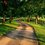# [Polynomials] A Case of a Non-Negative Polynomial that cannot be written as a Sum of Squares

Let's show that the Motzkin polynomial cannot be expressed as the sum of squares. Assume for the sake of contradiction, that $M(x,y) = x^4y^2+x^2y^4 +1-3x^2y^2 = \sum_{j=1}^{p} f_j (x,y)^2$$=\sum (A_j+B_jx+C_jy+D_jx^2+E_jxy+F_jy^2+G_jx^3+H_jx^2y+I_jxy^2+J_jy^3)^2,$

where $A_j,B_j,..., I_j, J_j$ are all real constants. By comparing the above expression to Motzkin's polynomial, we see that some of the coefficients in $\sum f_j (x,y)^2$ equal zero, because Motzkin's polynomial does not contain some terms in $\sum f_j(x,y)^2.$

Firstly, Motzkin's polynomial does not have $x^6, y^6$, so we can eliminate the constants $G_j$ and $J_j$, that is, $G_j=J_j=0$.

So now $f_j(x,y)= A_j+ B_jx + C_jy + D_jx^2 + E_jxy + F_jy^2 + H_jx^2y + I_jxy^2.$

Additionally, Motzkin's polynomial does not have the terms $x^4, y^4$, so we know that $D_j=F_j=0$.

So now $f_j(x,y)= A_j + B_jx + C_jy + E_jxy + H_jx^2y + I_jxy^2.$

Likewise, there is no $x^2$ term in the Motzkin polynomial, which means that $\sum B_j^2x^2=0$, hence each $B_j=0$. Finally,there is no $y^2$ term, so the coefficient of $y^2$ of $\sum f_j(x,y)^2$ is zero, so $\sum C_j^2 =0$ and each $C_j=0.$

Therefore, we are left with $f_j(x,y)= A_j + E_jxy + H_jx^2y + I_jxy^2,$ where $A_j, E_j, H_j, I_j$ are constants.

Following this procedure, we see that the coefficient of the $x^2y^2$ term in the Motzkin polynomial is -3. This means that

$\sum 2F_jD_j+E_j^2+2B_jI_j+2C_jH_j = \sum (2F_jD_j+E_j^2)^2 = -3.$

But if we take a look at $D_j$, we see that there is no $x^4$ term in the Motzkin polynomial, so $\sum D_j^2+2B_jG_j=0$. But we found above that $B_j=0$, so $\sum D_j^2=0$, hence each $D_j=0$. Therefore, $\sum (2F_jD_j+E_j^2) = \sum E_j^2 =-3.$

Since the sum of all the $E_j^2$ terms cannot be negative, this is a contradiction.

Hence we conclude that the Motzkin polynomial, $M(x,y) = x^4y^2+x^2y^4 +1-3x^2y^2$, is an example of a non-negative polynomial of two variables that cannot be written as the sum of squares.

Previously, we showed that the Motzkin polynomial is non-negative using the AM-GM inequality. This polynomial caught our interest, because it is non-negative in $\mathbb{R}^2$, but cannot be expressed as the sum of two squares of polynomials. The Motzkin polynomial illustrates that although a non-negative polynomial in one variable can always be expressed as the sum of squares, this is not necessarily the case for a polynomial in two variables.Note by Bright Glow
3 years, 1 month ago

This discussion board is a place to discuss our Daily Challenges and the math and science related to those challenges. Explanations are more than just a solution — they should explain the steps and thinking strategies that you used to obtain the solution. Comments should further the discussion of math and science.

When posting on Brilliant:

• Use the emojis to react to an explanation, whether you're congratulating a job well done , or just really confused .
• Ask specific questions about the challenge or the steps in somebody's explanation. Well-posed questions can add a lot to the discussion, but posting "I don't understand!" doesn't help anyone.
• Try to contribute something new to the discussion, whether it is an extension, generalization or other idea related to the challenge.
• Stay on topic — we're all here to learn more about math and science, not to hear about your favorite get-rich-quick scheme or current world events.

MarkdownAppears as
*italics* or _italics_ italics
**bold** or __bold__ bold
- bulleted- list
• bulleted
• list
1. numbered2. list
1. numbered
2. list
Note: you must add a full line of space before and after lists for them to show up correctly
paragraph 1paragraph 2

paragraph 1

paragraph 2

[example link](https://brilliant.org)example link
> This is a quote
This is a quote
    # I indented these lines
# 4 spaces, and now they show
# up as a code block.

print "hello world"
# I indented these lines
# 4 spaces, and now they show
# up as a code block.

print "hello world"
MathAppears as
Remember to wrap math in $$ ... $$ or $ ... $ to ensure proper formatting.
2 \times 3 $2 \times 3$
2^{34} $2^{34}$
a_{i-1} $a_{i-1}$
\frac{2}{3} $\frac{2}{3}$
\sqrt{2} $\sqrt{2}$
\sum_{i=1}^3 $\sum_{i=1}^3$
\sin \theta $\sin \theta$
\boxed{123} $\boxed{123}$

## Comments

There are no comments in this discussion.

×

Problem Loading...

Note Loading...

Set Loading...